# Chebyshev theorem

(diff) ← Older revision | Latest revision (diff) | Newer revision → (diff)

If a functionis continuous on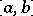and ifthen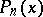is the polynomial of best uniform approximation for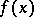, i.e.if and only if there exist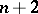points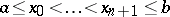in Chebyshev alternation, which means that the condition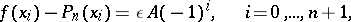is satisfied, where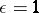or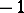. This theorem was proved by P.L. Chebyshev in 1854 (cf. ) in a more general form, namely for the best uniform approximation of functions by rational functions with fixed degrees of the numerator and denominator. Chebyshev's theorem remains valid if instead of algebraic polynomials one considers polynomialswhere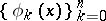is a Chebyshev system. The criterion formulated in Chebyshev's theorem leads to methods for the approximate construction of polynomials of best uniform (Chebyshev) approximation. In a somewhat different formulation Chebyshev's theorem can be extended to functions of a complex variable (cf. ) and to abstract functions (cf. ).